Many statistical modeling problems reduce to a minimization problem of the general form:

$\begin{equation} \mathrm{minimize}\subscript{\boldsymbol{\beta}\in\mathbb{R}^m}\quad f(\mathbf{X}, \boldsymbol{\beta}) + \lambda g(\boldsymbol{\beta}), \end{equation}$

or

$\begin{eqnarray} &\mathrm{minimize}\subscript{\boldsymbol{\beta}\in\mathbb{R}^m}\quad f(\mathbf{X}, \boldsymbol{\beta}),\\ &\mathrm{subject\,to}\quad g(\boldsymbol{\beta}) \leq t, \end{eqnarray}$

where $$f$$ is some type of loss function, $$\mathbf{X}$$ denotes the data, and $$g$$ is a penalty, also referred to by other names, such as “regularization term” (problems (1) and (2-3) are often equivalent by the way). Of course both, $$f$$ and $$g$$, may depend on further parameters.

There are multiple reasons why it can be helpful to check out the contours of such penalty functions $$g$$:

1. When $$\boldsymbol{\beta}$$ is two-dimensional, the solution of problem (2-3) can be found by simply taking a look at the contours of $$f$$ and $$g$$.
2. That builds intuition for what happens in more than two dimensions, and in other more general cases.
3. From a Bayesian point of view, problem (1) can often be interpreted as an MAP estimator, in which case the contours of $$g$$ are also contours of the prior distribution of $$\boldsymbol{\beta}$$.

Therefore, it is meaningful to visualize the set of points that $$g$$ maps onto the unit ball in $$\mathbb{R}^2$$, i.e., the set

$B\subscript{g} := \{ \mathbf{x}\in\mathbb{R}^2 : g(\mathbf{x}) \leq 1 \}.$

Below you see GIF images of such sets $$B\subscript{g}$$ for various penalty functions $$g$$ in 2D, capturing the effect of varying certain parameters in $$g$$. The covered penalty functions include the family of $$p$$-norms, the elastic net penalty, the fused penalty, the sorted $$\ell_1$$ norm, and several others.R code to reproduce the GIFs is provided.

## p-norms in 2D

First we consider the $$p$$-norm,

$g\subscript{p}(\boldsymbol{\beta}) = \lVert\boldsymbol{\beta}\rVert\subscript{p}^{p} = \lvert\beta\subscript{1}\rvert^p + \lvert\beta\subscript{2}\rvert^p,$

with a varying parameter $$p \in (0, \infty]$$ (which actually isn’t a proper norm for $$p < 1$$). Many statistical methods, such as LASSO (Tibshirani 1996) and Ridge Regression (Hoerl and Kennard 1970), employ $$p$$-norm penalties. To find all $$\boldsymbol{\beta}$$ on the boundary of the 2D unit $$p$$-norm ball, given $$\beta_1$$ (the first entry of $$\boldsymbol{\beta}$$), $$\beta_2$$ is easily obtained as

$\beta_2 = \pm (1-|\beta_1|^p)^{1/p}, \quad \forall\beta_1\in[-1, 1].$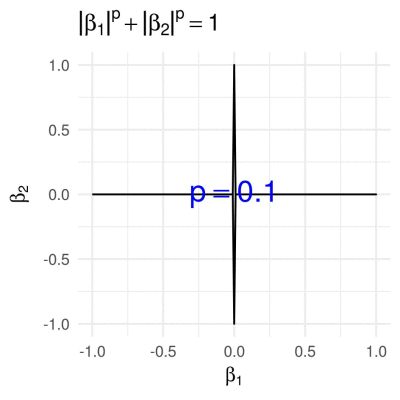## Elastic net penalty in 2D

The elastic net penalty can be written in the form

$g\subscript{\alpha}(\boldsymbol{\beta}) = \alpha \lVert \boldsymbol{\beta} \rVert\subscript{1} + (1 - \alpha) \lVert \boldsymbol{\beta} \rVert\subscript{2}^{2},$

for $$\alpha\in(0,1)$$. It is quite popular with a variety of regression-based methods (such as the Elastic Net, of course). We obtain the corresponding 2D unit “ball”, by calculating $$\beta\subscript{2}$$ from a given $$\beta\subscript{1}\in[-1,1]$$ as

$\beta\subscript{2} = \pm \frac{-\alpha + \sqrt{\alpha^2 - 4 (1 - \alpha) ((1 - \alpha) \beta\subscript{1}^2 + \alpha \beta\subscript{1} - 1)}}{2 - 2 \alpha}.$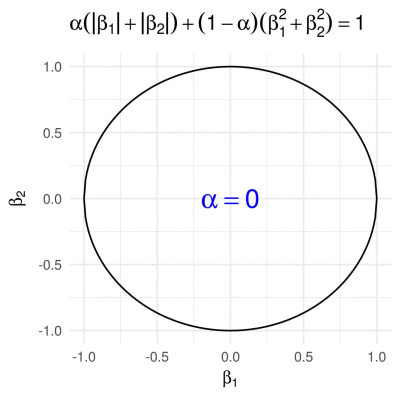## Fused penalty in 2D

The fused penalty can be written in the form

$g\subscript{\alpha}(\boldsymbol{\beta}) = \alpha \lVert \boldsymbol{\beta} \rVert\subscript{1} + (1 - \alpha) \sum\subscript{i = 2}^m \lvert \beta\subscript{i} - \beta\subscript{i-1} \rvert.$

It encourages neighboring coefficients $$\beta\subscript{i}$$ to have similar values, and is utilized by the fused LASSO (Tibshirani et. al. 2005) and similar methods.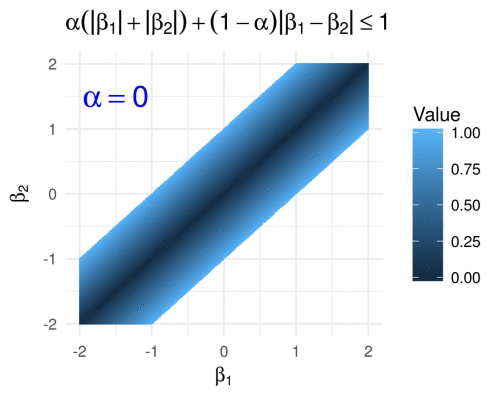(Here I have simply evaluated the fused penalty function on a grid of points in $$[-2,2]^2$$, because figuring out equations in parametric form for the above polygons was too painful for my taste…)

## Sorted L1 penalty in 2D

The Sorted $$\ell\subscript{1}$$ penalty is used in a number of regression-based methods, such as SLOPE (Bogdan et. al. 2015) and OSCAR (Bondell and Reich 2008). It has the form

$g\subscript{\boldsymbol{\lambda}}(\boldsymbol{\beta}) = \sum\subscript{i = 1}^m \lambda\subscript{i} \lvert \beta \rvert\subscript{(i)},$

where $$\lvert \beta \rvert\subscript{(1)} \geq \lvert \beta \rvert\subscript{(2)} \geq \ldots \geq \lvert \beta \rvert\subscript{(m)}$$ are the absolute values of the entries of $$\boldsymbol{\beta}$$ arranged in a decreasing order. In 2D this reduces to

$g\subscript{\boldsymbol{\lambda}}(\boldsymbol{\beta}) = \lambda\subscript{1} \max\{|\beta\subscript{1}|, |\beta\subscript{2}|\} + \lambda\subscript{2} \min\{|\beta\subscript{1}|, |\beta\subscript{2}|\}.$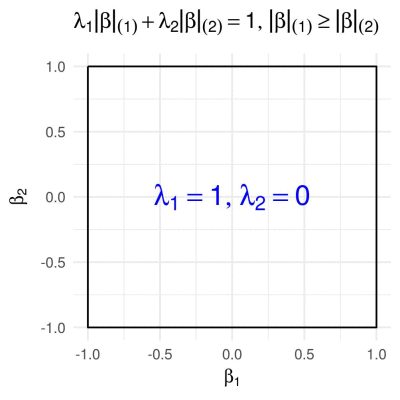## Difference of p-norms

It holds that

$\lVert \boldsymbol{\beta} \rVert\subscript{1} \geq \lVert \boldsymbol{\beta} \rVert\subscript{2},$

or more generally, for all $$p$$-norms it holds that

$(\forall p \leq q) : \lVert \boldsymbol{\beta} \rVert\subscript{p} \geq \lVert \boldsymbol{\beta} \rVert\subscript{q}.$

Thus, it is meaningful to define a penalty function of the form

$g\subscript{\alpha}(\boldsymbol{\beta}) = \lVert \boldsymbol{\beta} \rVert\subscript{1} - \alpha \lVert \boldsymbol{\beta} \rVert\subscript{2},$

for $$\alpha\in[0,1]$$, which results in the following.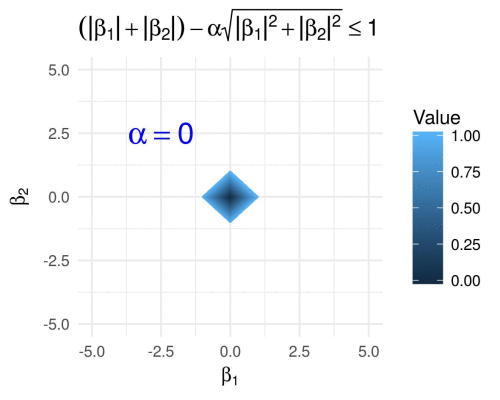We visualize the same for varying $$p \geq 1$$ fixing $$\alpha = 0.6$$, i.e., we define

$g\subscript{\alpha}(\boldsymbol{\beta}) = \lVert \boldsymbol{\beta} \rVert\subscript{1} - 0.6 \lVert \boldsymbol{\beta} \rVert\subscript{p},$

and we obtain the following GIF.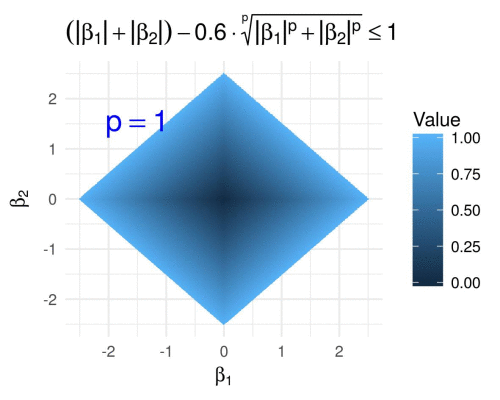## Hyperbolic tangent penalty in 2D

The hyperbolic tangent penalty, which is for example used in the method of variable selection via subtle uprooting (Su, 2015), has the form

$g\subscript{a}(\boldsymbol{\beta}) = \sum\subscript{i = 1}^p \tanh(a \beta\subscript{i}^2), \quad a>0.$

Contrary to all of the previously shown penalty functions, for any fixed $$a$$ the hyperbolic tangent penalty has round contours (for small values of $$g\subscript{a}$$) as well as contours with sharp corners (for larger values of $$g\subscript{a}$$).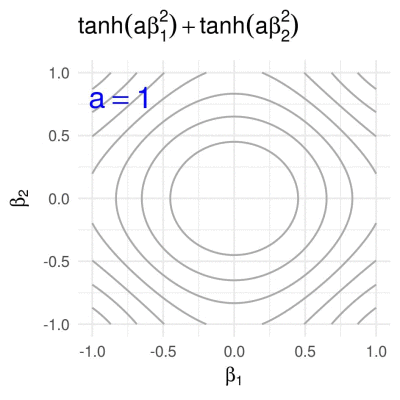# Code

The R code uses the libraries dplyr for data manipulation, ggplot2 for generation of figures, and magick to combine the individual images into a GIF.

Here are the R scripts that can be used to reproduce the above GIFs:

Should I come across other interesting penalty functions that make sense in 2D, then I will add corresponding further visualizations to the same Github repository.